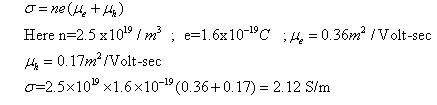Courses

# Test: Types Of Material Of Semiconductor

## 10 Questions MCQ Test Physics Class 12 | Test: Types Of Material Of Semiconductor

Description
This mock test of Test: Types Of Material Of Semiconductor for Class 12 helps you for every Class 12 entrance exam. This contains 10 Multiple Choice Questions for Class 12 Test: Types Of Material Of Semiconductor (mcq) to study with solutions a complete question bank. The solved questions answers in this Test: Types Of Material Of Semiconductor quiz give you a good mix of easy questions and tough questions. Class 12 students definitely take this Test: Types Of Material Of Semiconductor exercise for a better result in the exam. You can find other Test: Types Of Material Of Semiconductor extra questions, long questions & short questions for Class 12 on EduRev as well by searching above.
QUESTION: 1

### When a pure semiconductor is heated, its resistance

Solution:

As temperature increase electrons from valence band goes to the conduction band, so conductivity increases hence resistance decreases.

QUESTION: 2

### What is the order of forbidden gap in the energy bands of silicon?​

Solution:

Silicon has forbidden gap of 1.1 eV at 300 K temperature.

QUESTION: 3

### The densities of electrons and holes in an extrinsic semiconductor are 8 x 1015 cm-3and 5 x 1012 cm-3 respectively. The mobilities of electrons and holes are respectively 23 x 103 cm3 / Vs and 102 cm3 / Vs. Which type of semiconductor is it?​

Solution:

It is N- type semiconducter, because density of electrons is more here.

QUESTION: 4

A semiconductor is formed by

Solution:

The electrons surrounding each atom in a semiconductor are part of a covalent bond. A covalent bond consists of two atoms "sharing" a single electron. Each atom forms 4 covalent bonds with the 4 surrounding atoms. Therefore, between each atom and its 4 surrounding atoms, 8 electrons are being shared.

QUESTION: 5

Arrange the materials in ascending order of energy band gaps

Solution:

In the case of insulators, the energy gap is very large while in case of conductors energy gap is very short.This energy gap refers to the energy difference between valence band and conduction band whereas in semiconductors, this band gap value is in between that of conductors and insulators.

QUESTION: 6

In a semiconductor, current conduction is due

Solution:

There are two types of semiconductors-p type and n type. Therefore holes and electrons conduct current respectively

QUESTION: 7

Electrons moving like molecules of a gas exist in:

Solution:

Electrons in metal behave as gas.

QUESTION: 8

What is the value of dielectric constant of a air ?

Solution:

The Dielectric Constant (E) is always less than or equal to Eo, So the dielectric constant is greater than or equal to 1. The larger the dielectric constant, the more charge can be stored.

QUESTION: 9

Mobilities of electrons and holes in a sample of intrinsic germanium semiconductor at room temperature are 0.36m2/volt-sec and 0.17 m2/volt-sec respectively. If the electron and hole densities are each equal to 2.5 X 1019/m3, the conductivity is.​

Solution:QUESTION: 10

In a semiconductor, the forbidden energy gap between valence band and conduction band is about​

Solution:

In semiconductors, the forbidden gap between valence band and conduction band is very small. It has a forbidden gap of about 1 electron volt (eV)